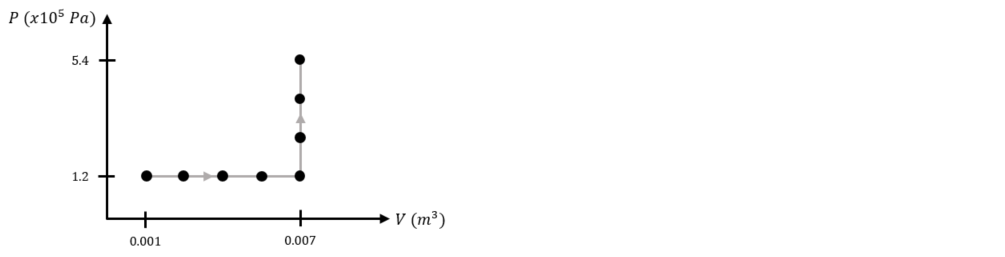Problem: Two different experiments were carried out on 5 kg of an ideal gas with a molar mass of 100 g/mol, the results of which are shown in the following figure. For the entire experiment, heat was added into the ideal gas at a rate of 100 J/s, and each successive data point in the figure is 1 ms apart.(a) What is the specific heat at constant volume? What is the heat capacity at constant volume?(b) What is the specific heat at constant pressure? What is the heat capacity at constant pressure?

FREE Expert Solution
94% (150 ratings)
Problem Details

Two different experiments were carried out on 5 kg of an ideal gas with a molar mass of 100 g/mol, the results of which are shown in the following figure. For the entire experiment, heat was added into the ideal gas at a rate of 100 J/s, and each successive data point in the figure is 1 ms apart.

(a) What is the specific heat at constant volume? What is the heat capacity at constant volume?

(b) What is the specific heat at constant pressure? What is the heat capacity at constant pressure?